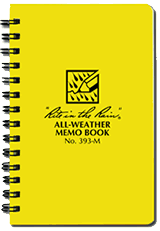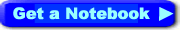Home » Free Printables » Printable Graph Paper » Math Graph Paper

# Math Graph Paper - Axis Graph Paper

### What is Math Graph Paper?

Math graph paper, also known as "axis graph paper" or "Cartesian coordinates paper," is a piece of graph paper with a pre-drawn X and Y axis. The origin of the graph is positioned at the center of the paper. This type of graph paper is most often used in math assignments for graphing functions through a series of negative and positive numbers.

This type of graphing helps students understand the shape of a line or curve associated with an equation or function. Many math teachers believe that students who produce a few graphs by hand before using a computer program obtain a deeper understanding of equations and graphs.

### About our Math Graph Paper

We have two basic math axis papers, one with black lines and one with blue. Choose the paper that will contrast with the pencil or pen color that you will be plotting with. These papers are provided as printable .pdf documents. They are designed to print on a standard sheet of 8 ½ x 11 paper. They can be opened and printed with a .pdf document reader such as Adobe Reader.Waterproof Graph Paper Notebooks
4 sizes available